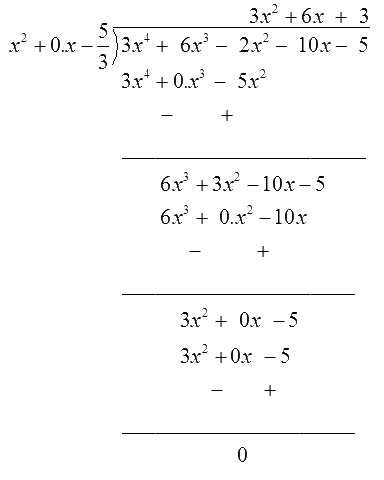# Ex.2.3 Q3 Polynomials Solution - NCERT Maths Class 10

Go back to  'Ex.2.3'

## Question

Obtain all other zeroes of

$$3{x^4} + {\text{ }}6{x^3}-{\text{ }}2{x^2}-{\text{ }}10x-{\text{ }}5,$$

if two of its zeroes are

\begin{align}\sqrt {\frac{5}{3}} \;{\text{and}}\; - \sqrt {\frac{5}{3}} \,.\end{align}

Video Solution
Polynomials
Ex 2.3 | Question 3

## Text Solution

What is known?

Two zeroes of a polynomial are \begin{align}\sqrt {\frac{5}{3}}\end{align} and \begin{align}-\sqrt {\frac{5}{3}}.\end{align}

What is unknown?

All other zeroes of

\begin{align}3 x^{4}+6 x^{3}-2 x^{2}-10 x-5\end{align}

Reasoning:

You can solve this question by following the steps given below

Given polynomial

$$p(x)=3 x^4+6 x^3-2 x^2-10 x-5$$

Two zeroes of the polynomial are given as \begin{align}\sqrt {\frac{5}{3}} \end{align} and \begin{align}- \sqrt {\frac{5}{3}} .\end{align}

Therefore,

\begin{align} & \left( {x - \sqrt {\frac{5}{3}} } \right)\left( {x + \sqrt {\frac{5}{3}} } \right) \\ \\ & = \left( {{x^2} - \sqrt {\frac{5}{3}} } \right)\end{align}

is a factor of

$$\,3{x^4} + 6{x^3} - 2{x^2} - 10x - 5.$$

Divide $$3{x^4} + 6{x^3} - 2{x^2} - 10x - 5$$ by \begin{align}\left( {{x^2} - \frac{5}{3}} \right)\end{align}

On dividing you will get the quotient and the remainder.

Put the values of dividend, divisor, quotient and remainder in the division algorithm.

Now you can easily find out the other zeroes of the polynomial.

Steps:

$$P\left( x \right)= \begin{bmatrix} 3{x^4} + 6{x^3}-2{x^2} \\ -10x-5 \end{bmatrix}$$

Since, the two zeroes are \begin{align}\sqrt {\frac{5}{3}\,}\end{align} and \begin{align}- \sqrt {\frac{5}{3}} .\end{align}

Therefore,

\begin{align} & \left( {x- \sqrt {\frac{5}{3}} } \right) \left( {x + \sqrt {\frac{5}{3}} } \right) \\ \\ & = \left( {{x^2}-\sqrt {\frac{5}{3}} } \right)\end{align}

is a factor of

\begin{align}3{x^4} + {\text{ }}6{x^3}-{\text{ }}2{x^2}-{\text{ }}10x-{\text{ }}5.\end{align}

Therefore, we divide the given polynomial by \begin{align}{x^2} - \frac{5}{3}\end{align}.Therefore

\begin{align} &3{x^4} + 6{x^3}-2{x^2}-10x-5\\ &=\!\left( {{x^2} - \frac{5}{3}} \right)\left( {3{x^2} + {\text{ }}6x + 3} \right)\!+\!0\\ &= 3\left( {{x^2} - \frac{5}{3}} \right)\left( {{x^2} + 2x + 1} \right)\end{align}

We factorize

${{x}^{2}}+\text{ }2x~+\text{ }1~={{\left( ~~x~+\text{ }1~ \right)}^{2}}$

Therefore, its zero is given by

$x+1= 0,\quad x = - 1$

As it has the term $${{\left( x+ 1 \right)}^{2}}.$$

Therefore, there will be two identical zeroes at $$x = - 1.$$

Hence the zeroes of the given polynomial are \begin{align}\sqrt {\frac{5}{3}} , - \sqrt {\frac{5}{3}} ,\, - 1\,\end{align} and \begin{align}- 1.\end{align}

Learn from the best math teachers and top your exams

• Live one on one classroom and doubt clearing
• Practice worksheets in and after class for conceptual clarity
• Personalized curriculum to keep up with school